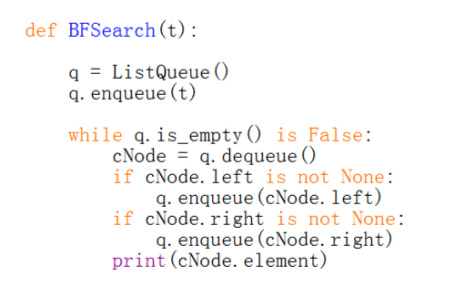## 1 Algorithm 算法

• An algorithm is a step-by-step procedure for performing some task in a finite amount of time.

### 1.1 Principle of algorithm analysis

How do we define a“good” algorithm?

• Running time is a natural measure of “goodness,” since time is a precious resource—computer solutions should run as fast as possible
• Space usage is another major issue to consider when we design an algorithm, since we only have limited storage spaces

Measuring the running time:

1) Counting primitive operations

To analyse the running time of an algorithm without performing experiments, we perform an analysis directly on a high-level description of the algorithm

primitive operations:

• Assigning an identifier to an object
• Determining the object associated with an identifier
• Performing an arithmetic operation (for example, adding two numbers)
• Comparing two numbers
• Accessing a single element of a Python list by index
• Calling a function (excluding operations executed within the function)
• Returning from a function.

2) Measuring Operations as a Function of Input Size

To capture the order of growth of an algorithm’s running time, we will associate, with each algorithm, a function `f(n)` that characterizes the number of primitive operations that are performed as a function of the input size n.

3) Focusing on the Worst-Case Input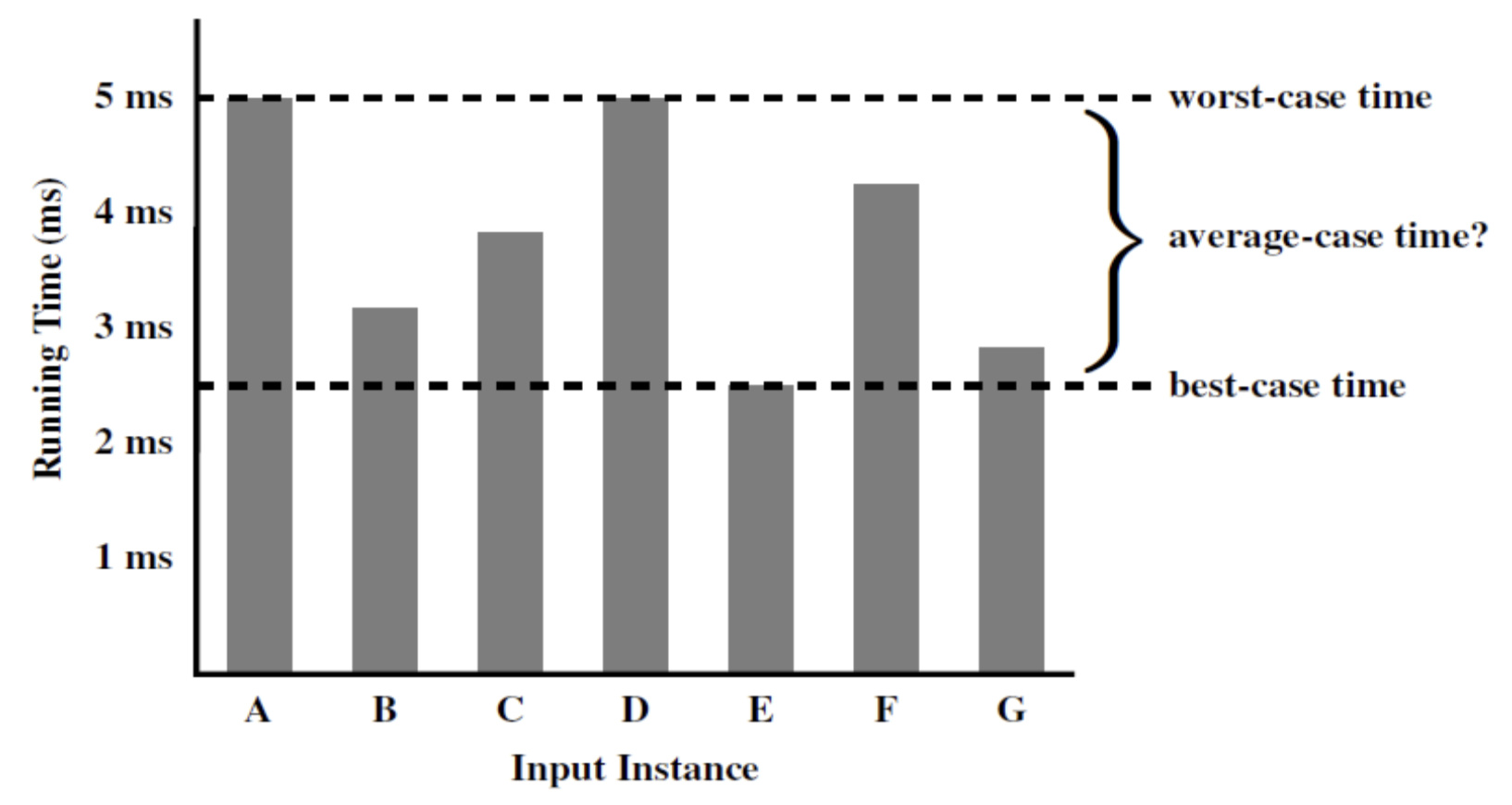### 1.2 algorithm analysis

1) The 7 functions used in algorithm analysis

We may use the following 7 functions to measure the time complexity of an algorithm:

• constant
• logarithm
• linear
• N-log-N
• quadratic
• cubic and other polynomials
• exponential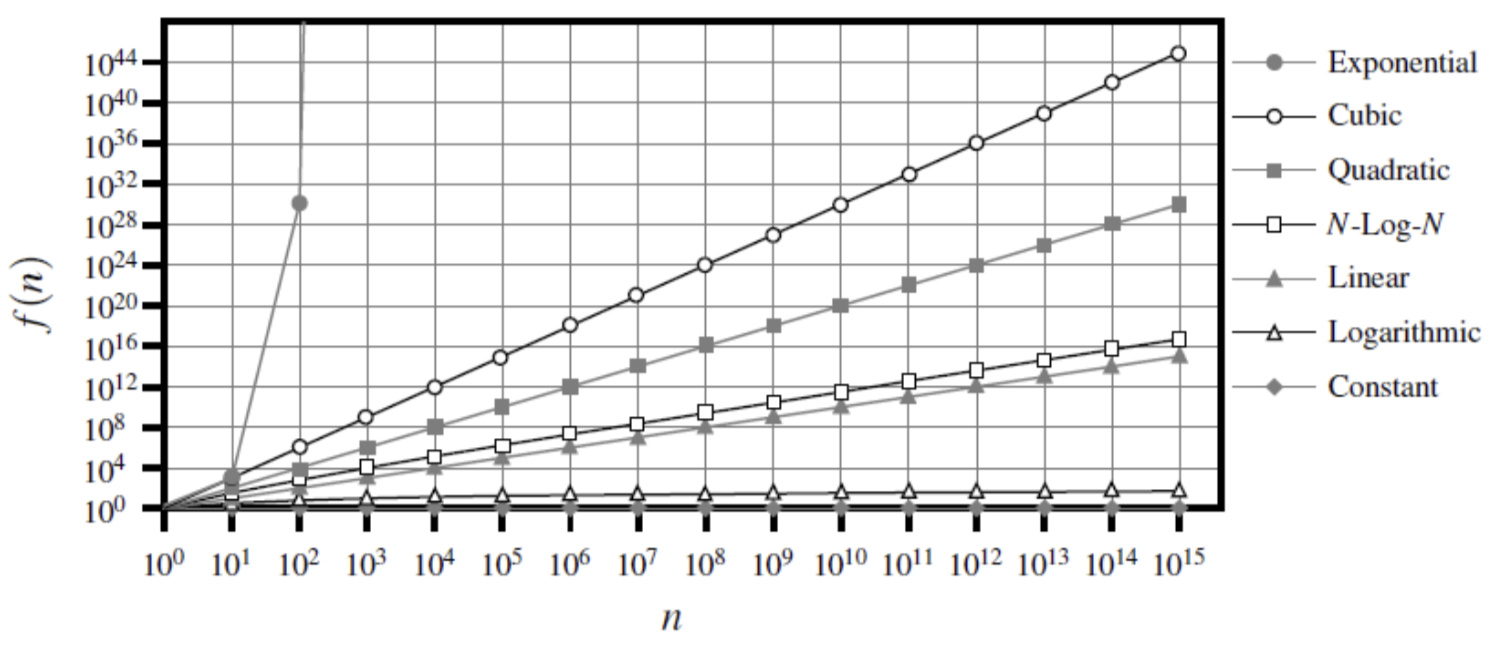2) Asymptotic Analysis 渐进分析

• In algorithm analysis, we focus on the growth rate of the running time as a function of the input size n, taking a “big-picture” approach
• Vocabulary for the analysis and design of algorithms
• “Sweet spot” for high-level reasoning about algorithms
• Coarse enough to supress unnecessary details, e.g. architecture/language/compiler…
• Sharp enough to make meaningful comparisons between algorithms

3) The big Oh notation

• Let `f(n)` and `g(n)` be functions mapping positive integers to positive real numbers.
• We say that `f(n)` is `O(g(n))` if there is a real constant `c > 0` and an integer constant `n0 ≥ 1` such that
`f(n) ≤ cg(n)`, for `n ≥ n0`
• This definition is often referred to as the “big-Oh” notation
• Example: The function `8n+5` is `O(n)`, `g(n) = n` `c = 9`, `n0 = 10`

4) Some Properties of the Big-Oh Notation

The big-Oh notation allows us to ignore constant factors and lower-order terms and focus on the main components of a function that affect its growth.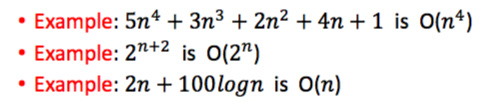In general, we should use the big-Oh notation to characterize a function as closely as possible

### 1.3 Comparative analysis

Q:
An algorithm A, which has a running time of ( O(n), and an algorithm B, which has a running time of O(𝑛 ). Which algorithm is better?

A:
Algorithm A is asymptotically (渐近) better than algorithm B, Constant of A may very large ,so it is not always better than b.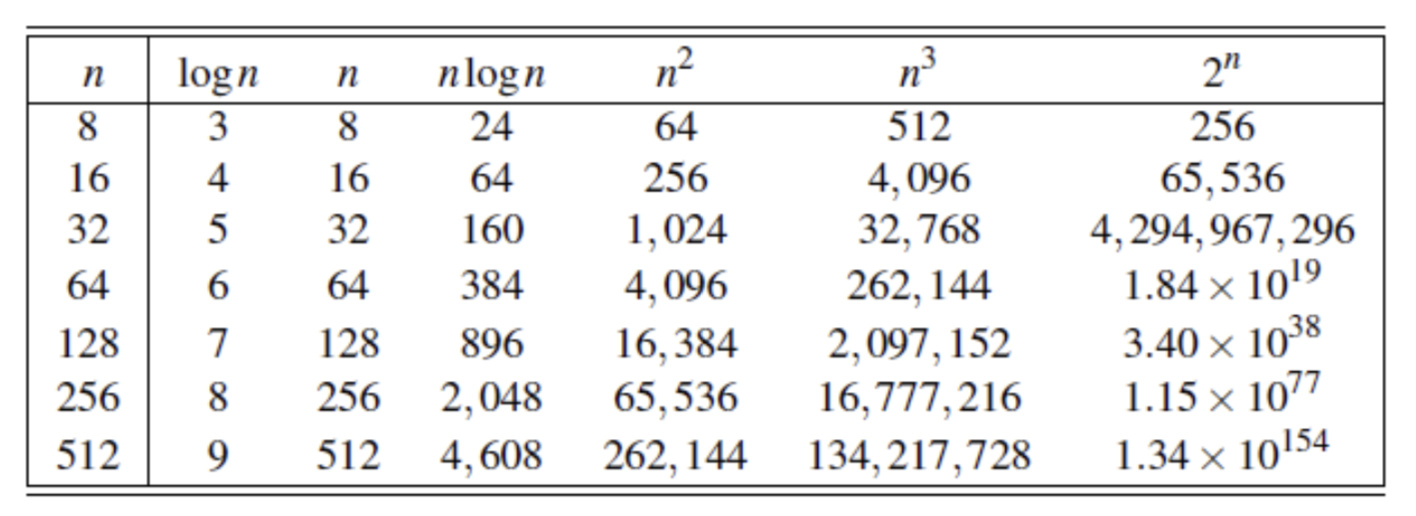1) The line of tractability

• To differentiate efficient and inefficient algorithms, the general line is between polynomial time algorithms and exponential time algorithms
• The distinction between polynomial-time and exponential- time algorithms is considered a robust measure of tractability

### 1.4 Recursion 递归

1) definition

• First, a recursive definition contains one or more base cases, which are defined non-recursively in terms of fixed quantities
• Second, it also contains one or more recursive cases, which are defined by appealing to the definition of the function being defined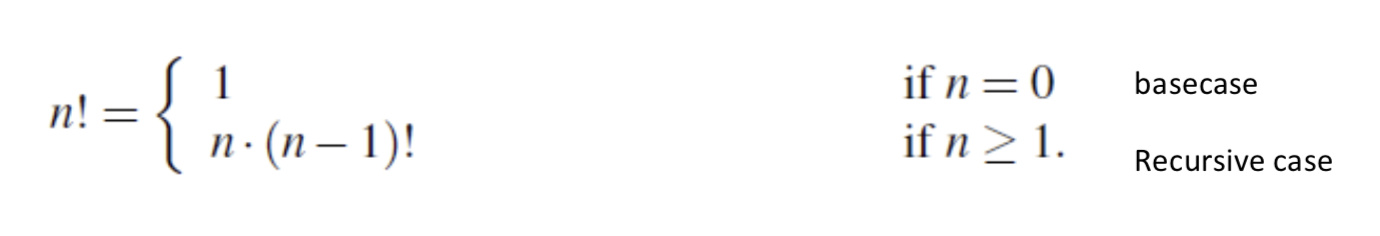2) How Python implements recursion

• In Python, each time a function (recursive or otherwise) is called, a structure known as an activation record or frame is created to store information about the progress of that invocation of the function
• This activation record stores the function call’s parameters and local variables (哪个函数被call了，函数的参数parametersxg，call这个函数的位置location)
• When the execution of a function leads to a nested function call, the execution of the former call is suspended（悬挂，暂停） and its activation record stores the place in the source code at which the flow of control should continue upon return of the nested call

3) The recursive trace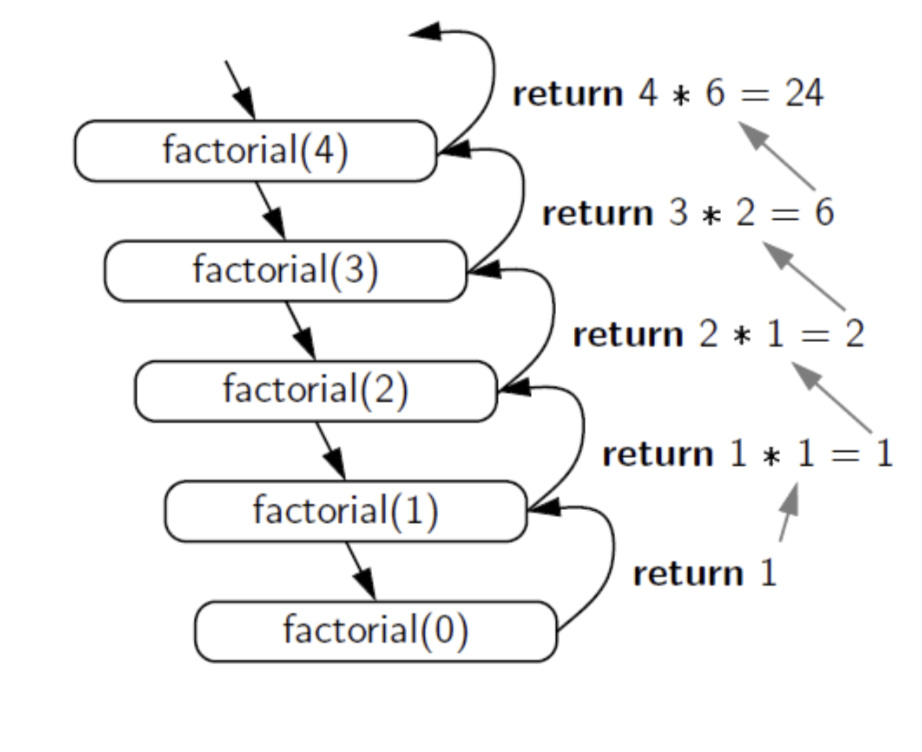4) 递归算法的通用结构：

### 1.5 Binary search 二分法

A classic and very useful recursive algorithm.

When the sequence is unsorted, the standard approach to search for a target value is to use a loop to examine every element, until either finding the target or exhausting the data set; This is known as the sequential search algorithm

Code: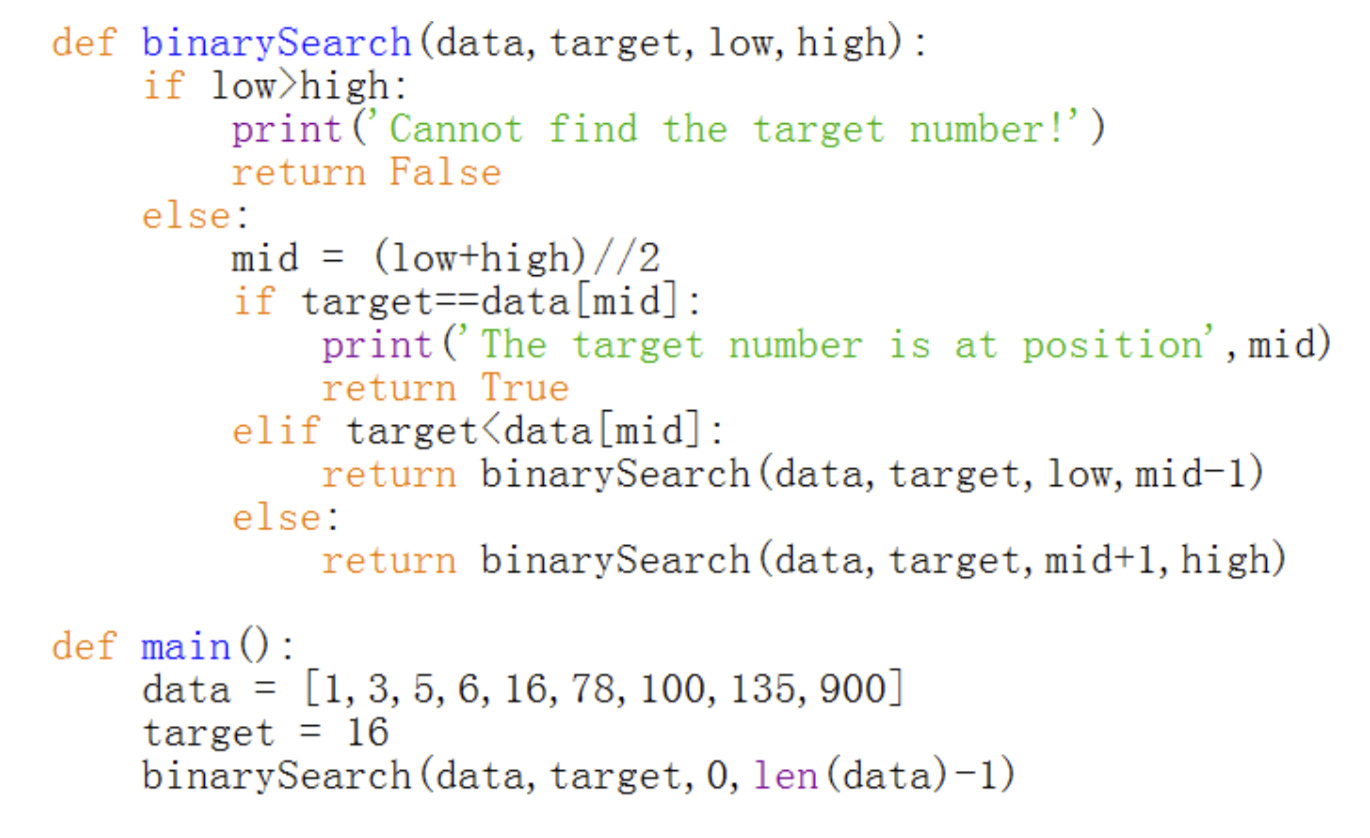Time complexity:

The binary search algorithm runs in `O(logn)` time for a sorted sequence with n elements.

`N *（1/2）^x = 1` —-> `x = logn`

### 1.6 Bubble sort 冒泡排序

Its general procedure is:

1. Iterate over a list of numbers, compare every element i with the following element i+1, and swap them if i is larger
2. Iterate over the list again and repeat the procedure in step 1, but ignore the last element in the list
3. Continuously iterate over the list, but each time ignore one more element at the tail of the list, until there is only one element left

Code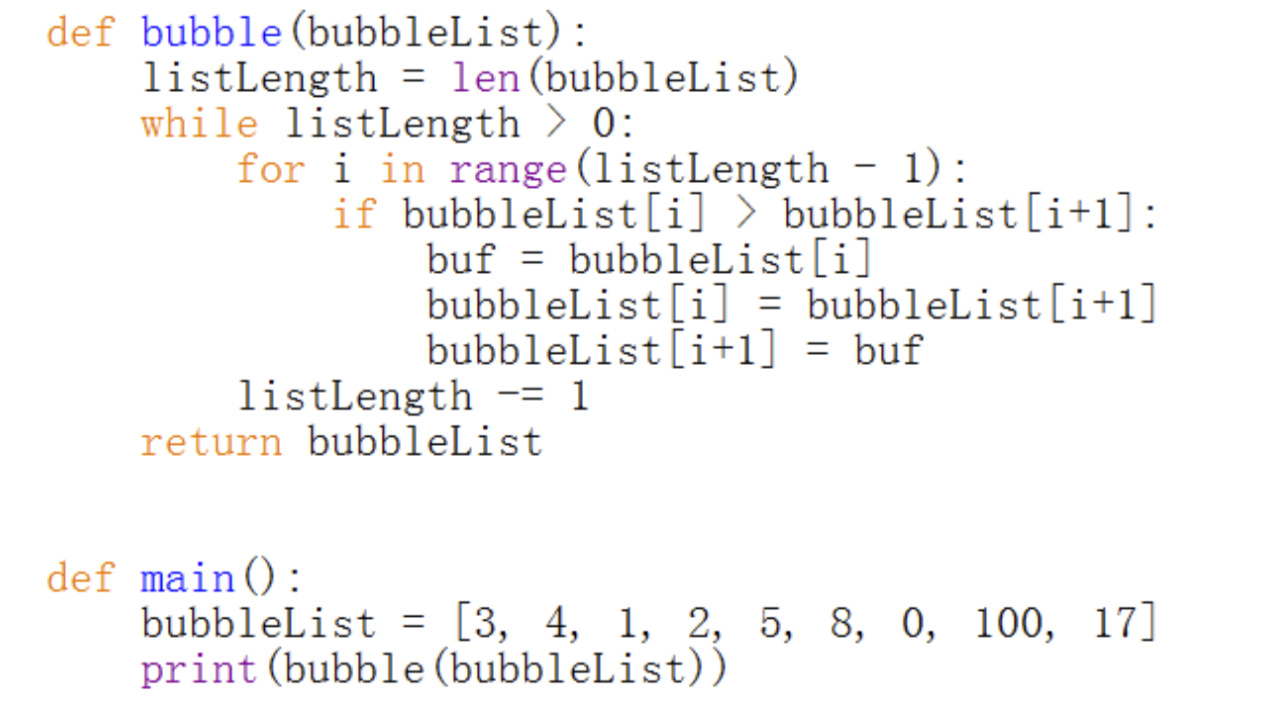### 1.7 Quick sort 快速排序

1) 分治思想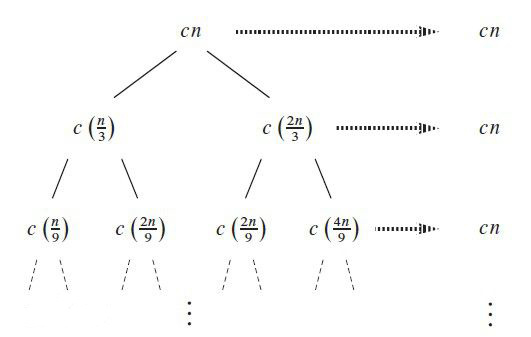2) 快速排序

• The main procedure of quick sort algorithm is:

1. Pick an element, called a pivot, from the array
2. Partitioning: reorder the array so that:
• all elements with values less than the pivot come before the pivot,

• while all elements with values greater than the pivot come after it

• (equal values can go either way).

After this partitioning, the pivot is in its final position. This is called the partition operation

1. Recursively 递归 apply the above steps to the sub-array of elements with smaller values and separately to the sub-array of elements with greater values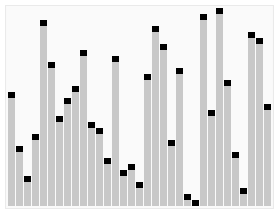## 2 Data structure 数据结构

• A data structure is a systematic way of organizing and accessing data

### 2.1 stack 堆栈

• A stack is a collection of objects that are inserted and removed according to the last-in, first-out (LIFO) principle.
• A user may insert objects into a stack at any time, but may only access or remove the most recently inserted object that remains (at the so-called “top” of the stack).

1) example

Web Browser

• Internet Web browsers store the addresses of recently visited sites in a stack. Each time a user visits a new site, that site’s address is “pushed” onto the stack of addresses. The browser then allows the user to “pop” back to previously visited sites using the “back” button.

Text editor

• Text editors usually provide an “undo” mechanism that cancels recent editing operations and reverts to former states of a document. This undo operation can be accomplished by keeping text changes in a stack.

2) The stack class

Generally, a stack may contain the following methods: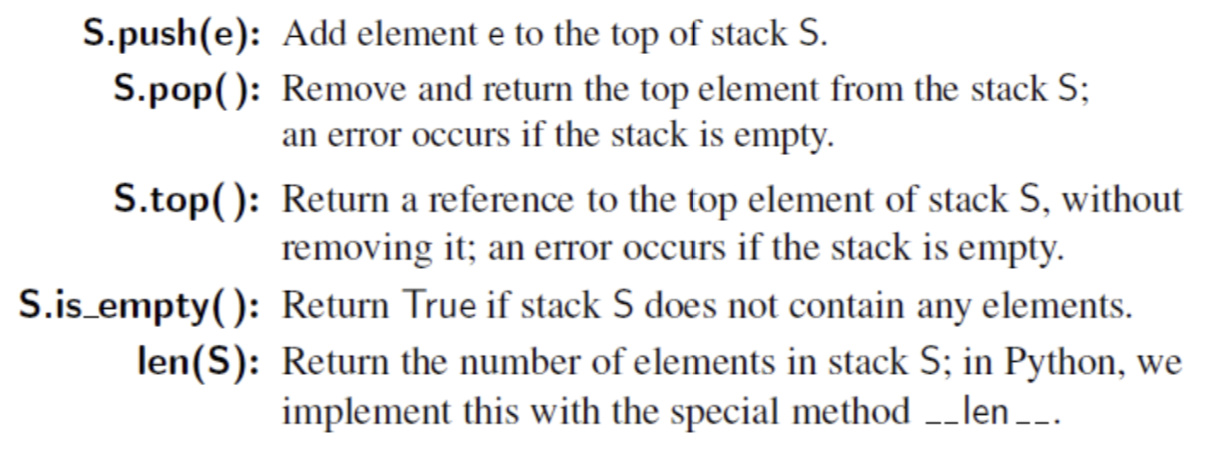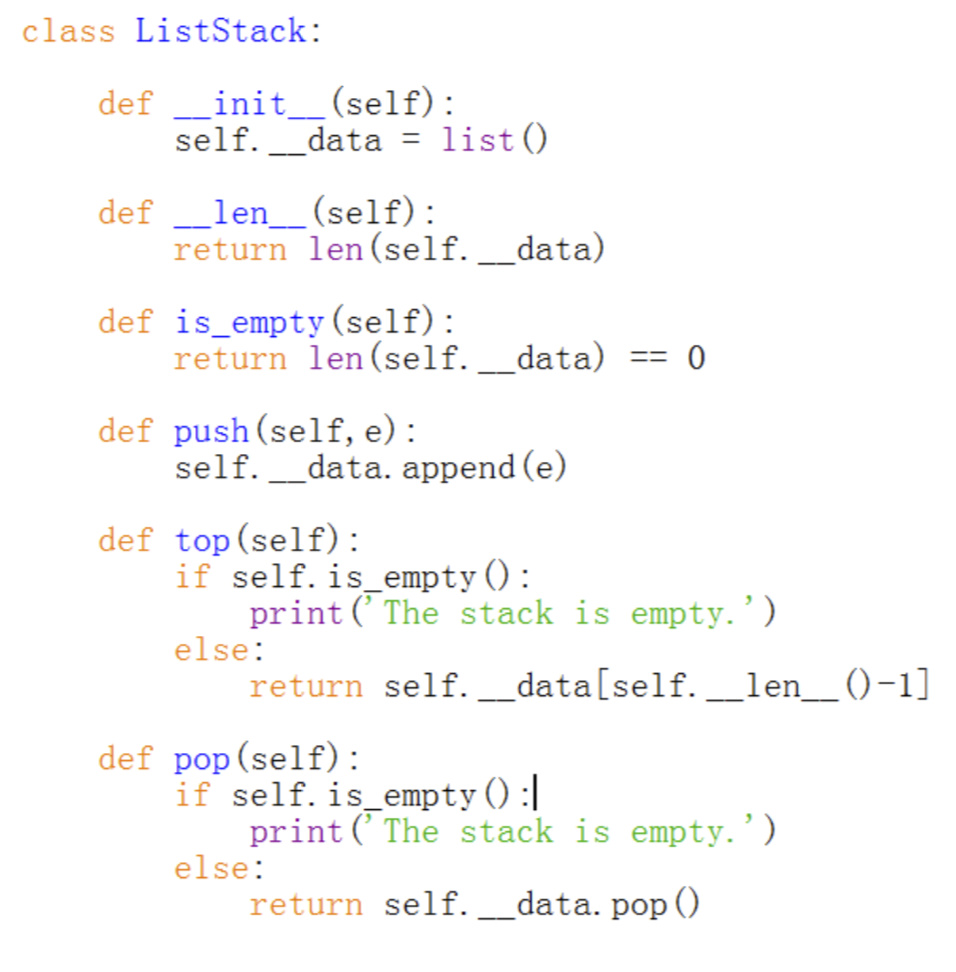3) Practice

1. Reverse a list using stack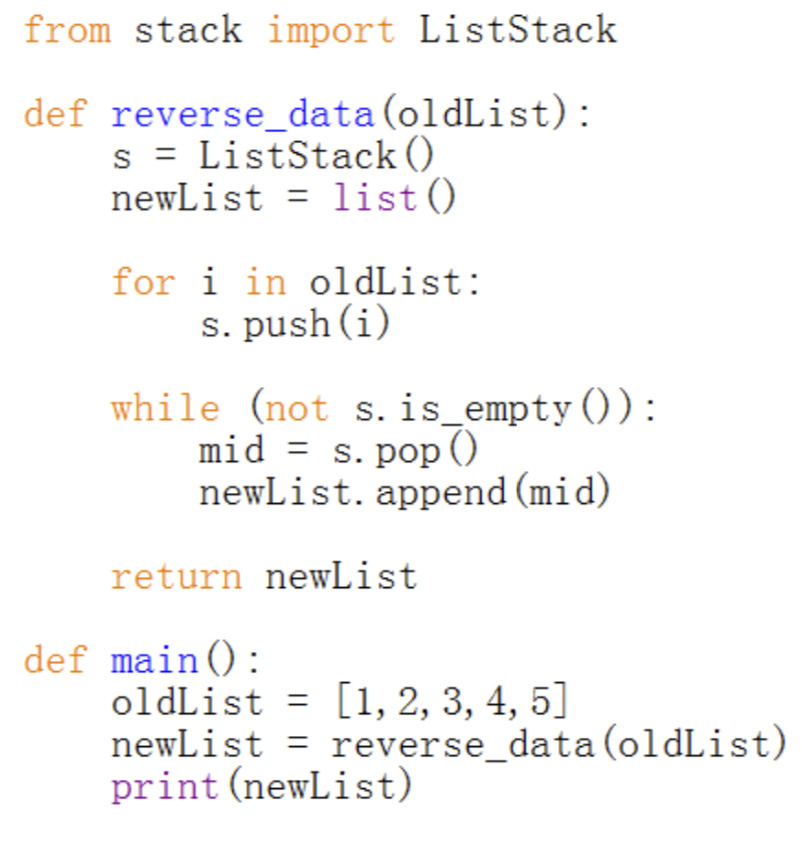1. Brackets match checking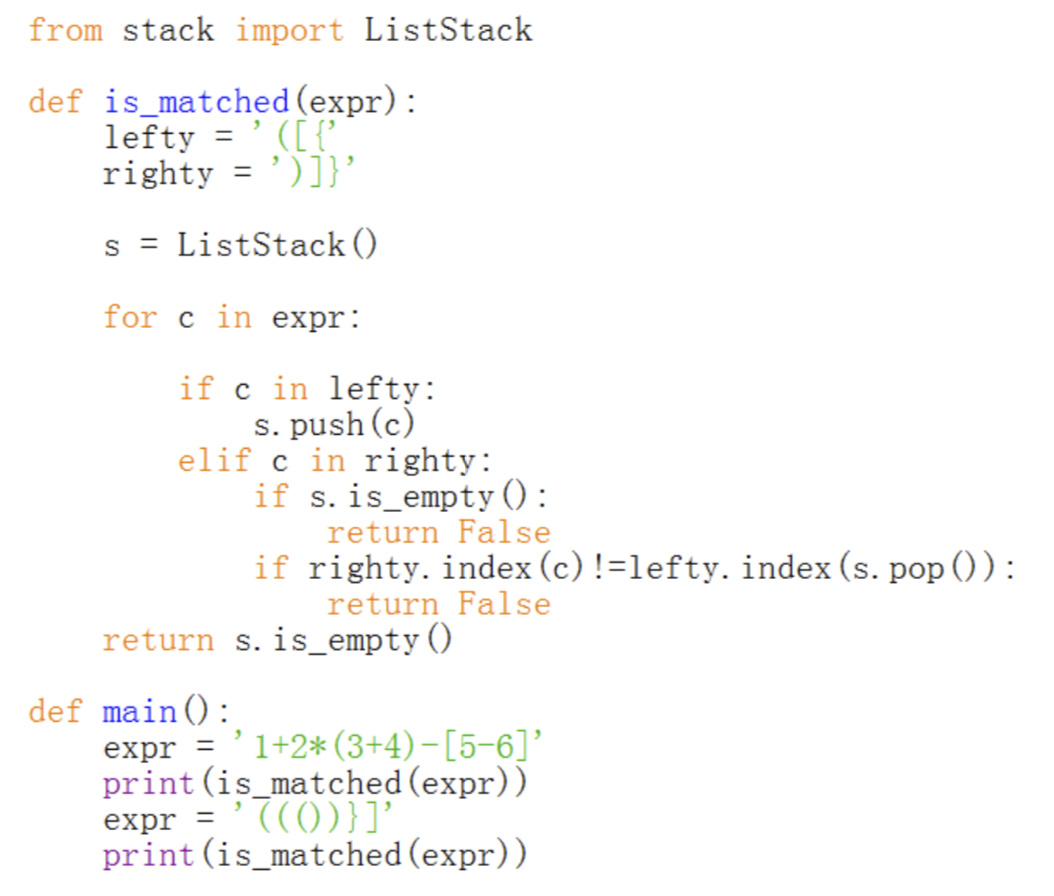1. Matching Tags in HTML Language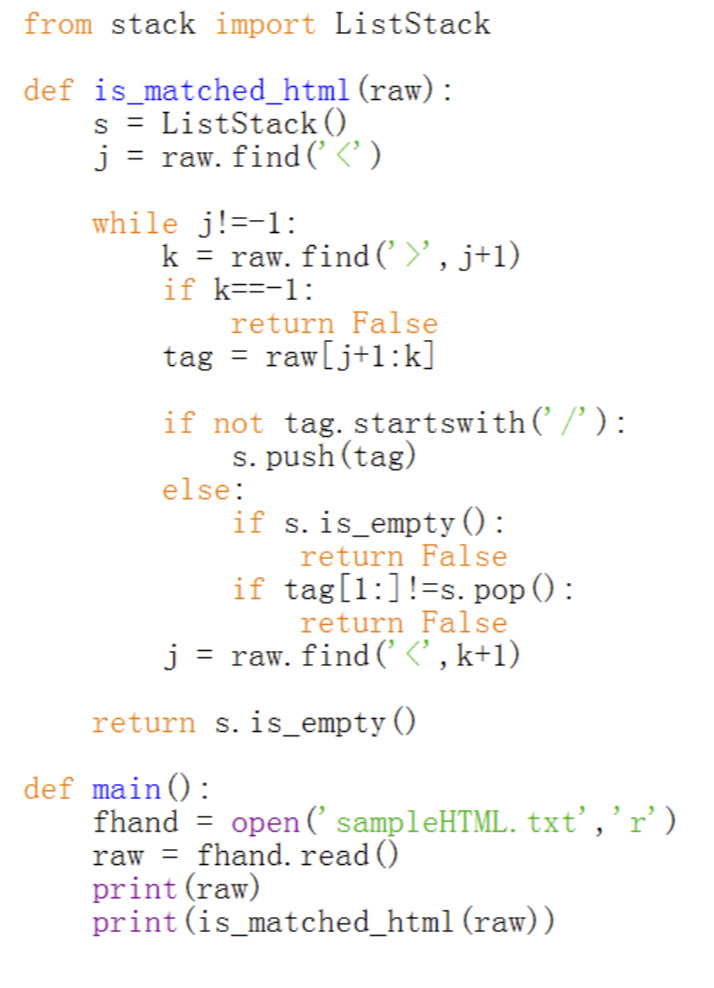### 2.2 Queue 队列

• Queue is another fundamental data structure
• A queue is a collection of objects that are inserted and
removed according to the first-in, first-out (FIFO) principle
• Elements can be inserted at any time, but only the element that has been in the queue the longest can be next removed

1) the queue class

The queue class may contain the following methods: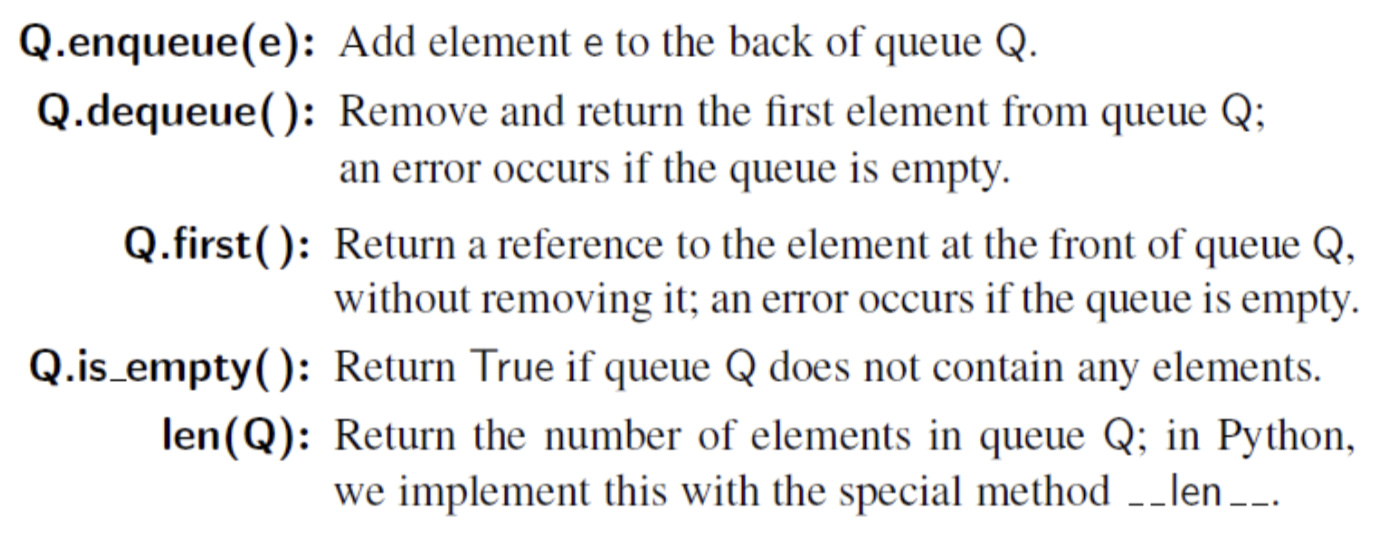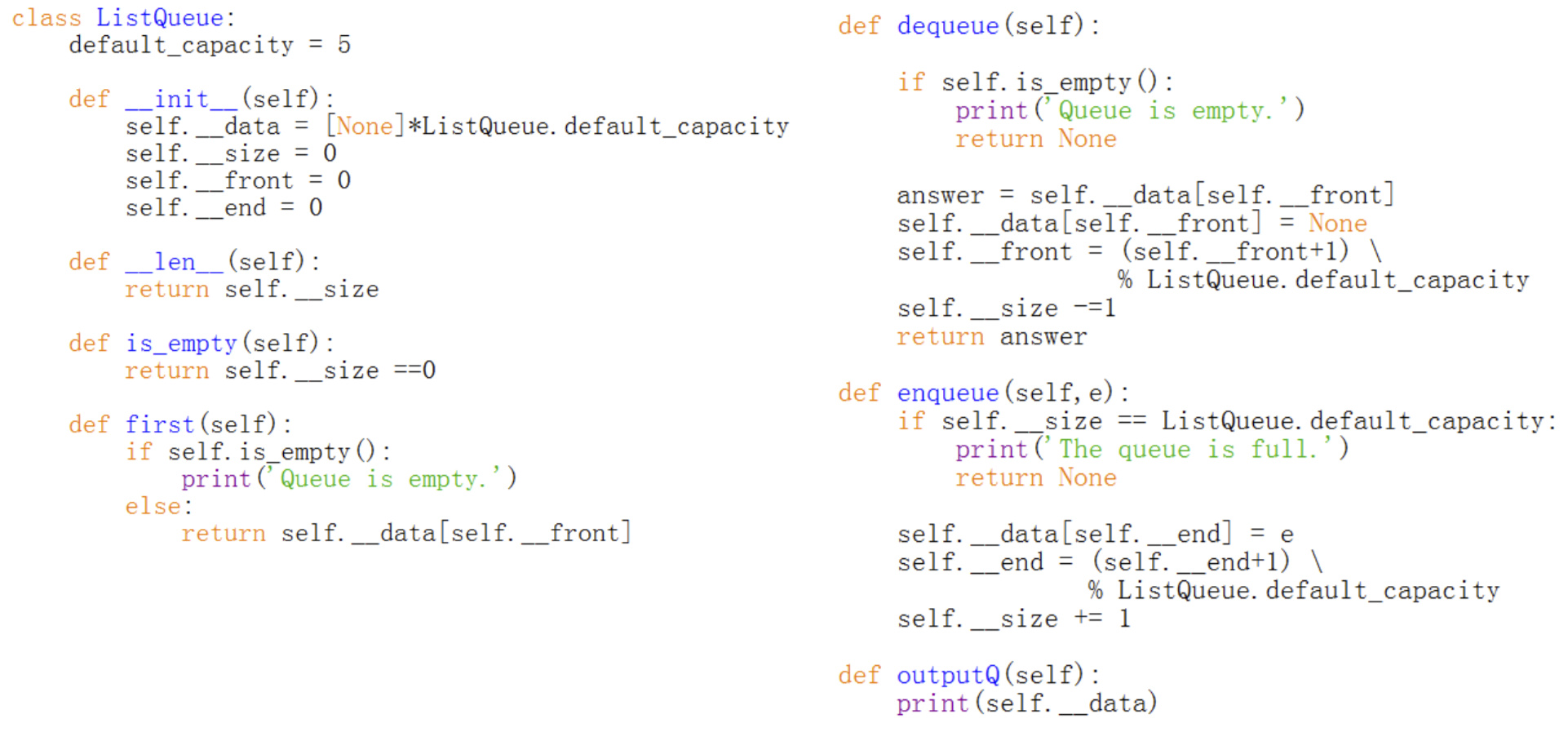2) Practice: Simulating a web service

An online video website handles service requests in the following way:

1. It maintains a service queue which stores all the unprocessed service requests.
2. When a new service request arrives, it will be saved at the end of the service queue.
3. The server of the website will process each service request on a “first-come-first-serve” basis.

### 2.3 Linked list 链表

1) list in python

• Python’s list class is highly optimized, and often a great choice for storage
• However, many programming languages do not support this kind of optimized list data type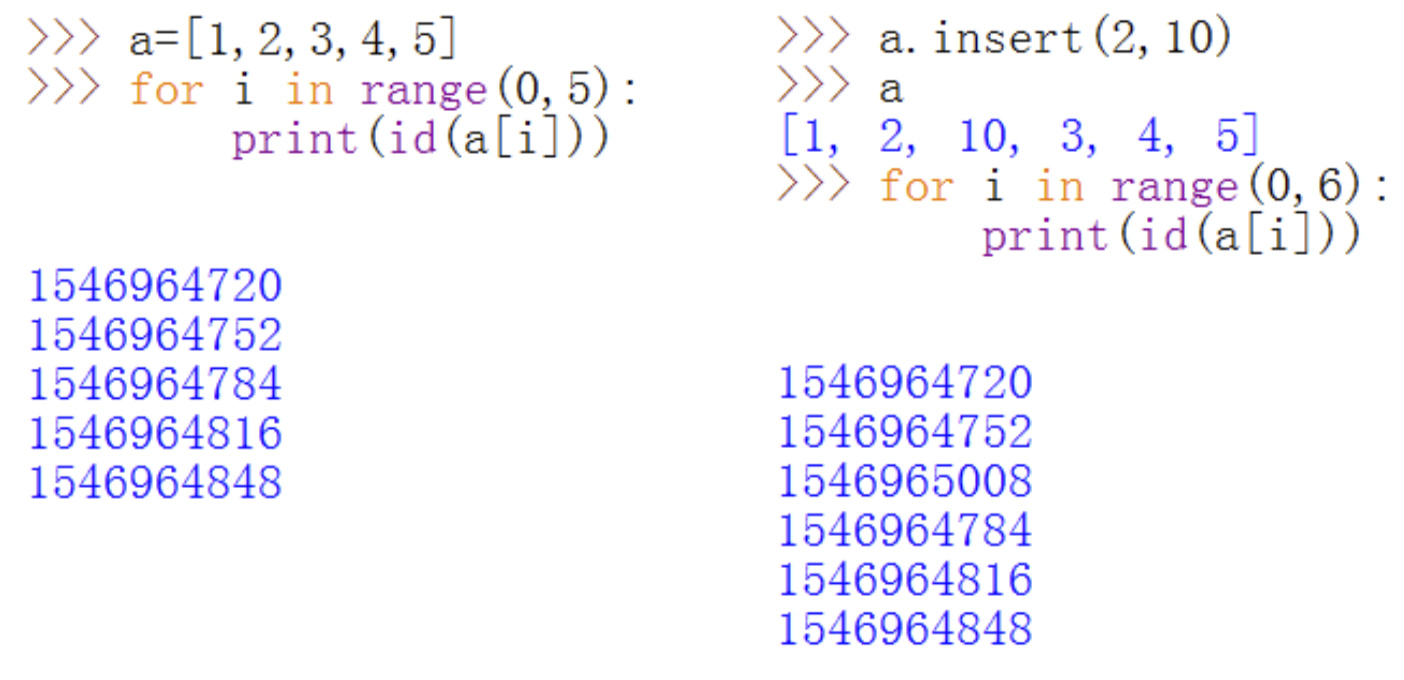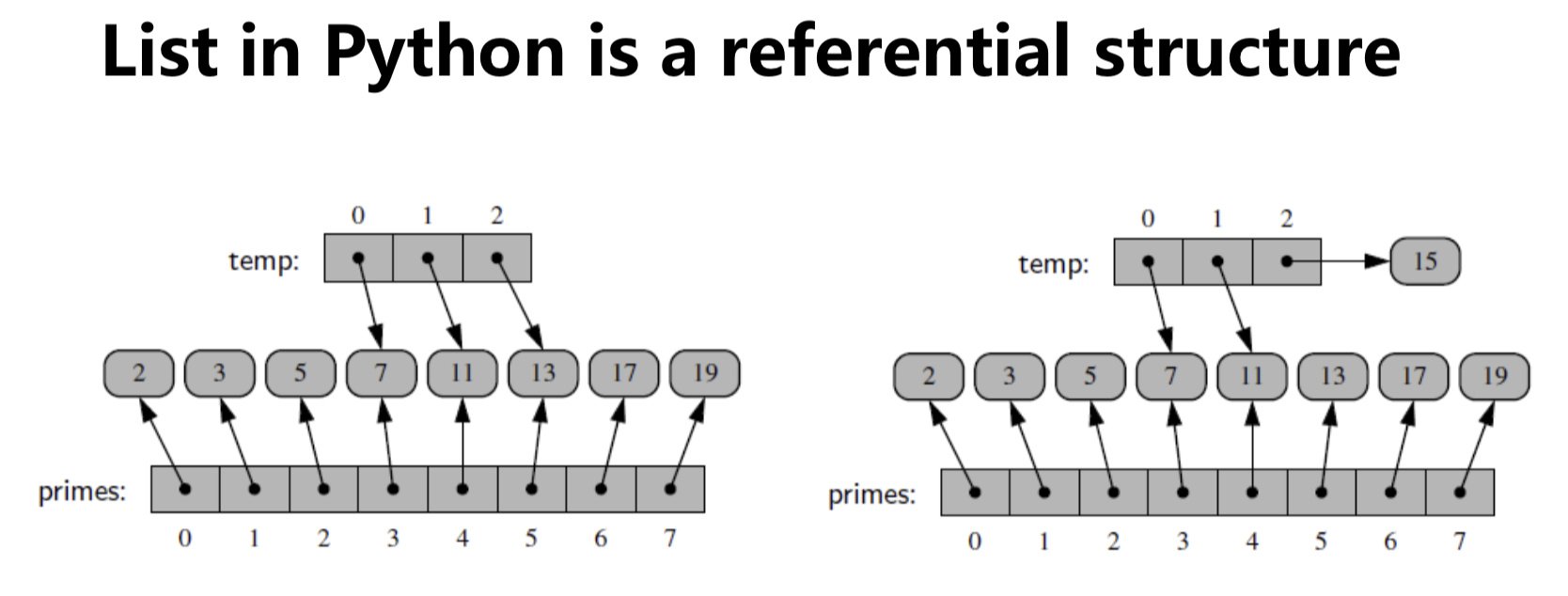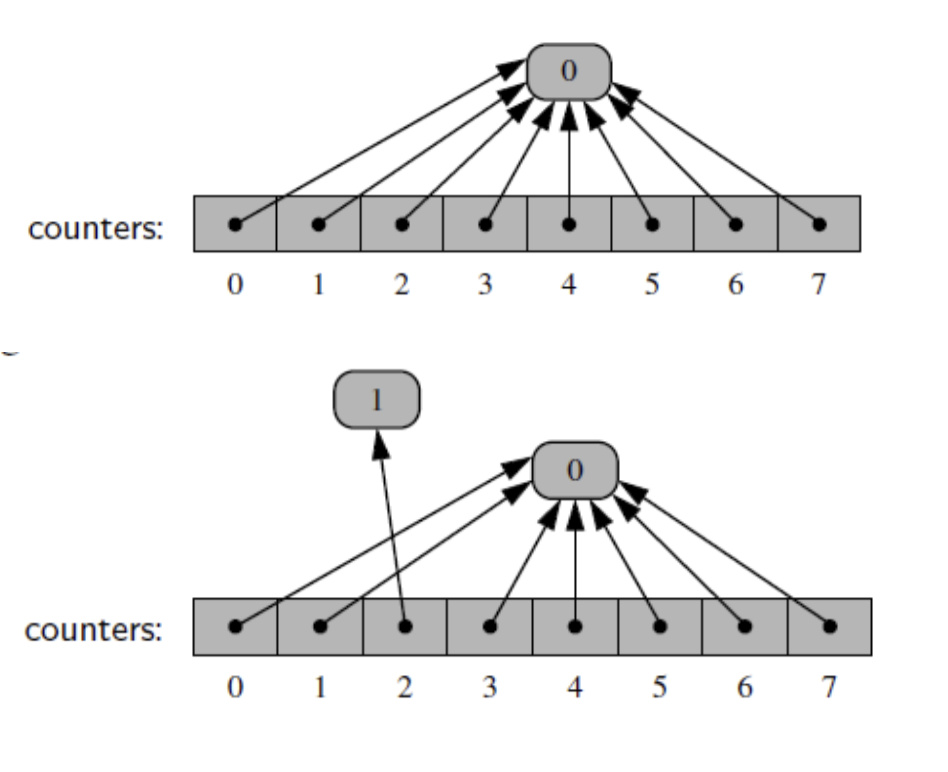2) Compact array

• A collection of numbers are usually stored as a compact array in languages such as C/C++ and Java
• A compact array is storing the bits that represent the primary data (not reference)
• The overall memory usage will be much lower for a compact structure because there is no overhead devoted to the explicit storage of the sequence of memory references (in addition to the primary data)

3) Linked List

• A singly linked list, in its simplest form, is a collection of nodes that collectively form a linear sequence
• Each node stores a reference to an object that is an element of the sequence, as well as a reference to the next node of the list

• The first and last nodes of a linked list are known as the head and tail of the list, respectively
• By starting at the head, and moving from one node to another by following each node’s next reference, we can reach the tail of the list
• We can identify the tail as the node having None as its next reference. This process is commonly known as traversing the linked list.
• Because the next reference of a node can be viewed as a link or pointer to another node, the process of traversing a list is also known as link hopping or pointer hopping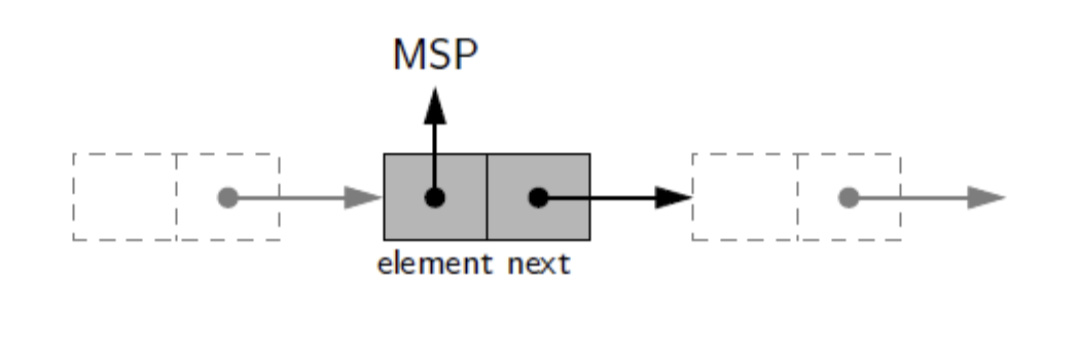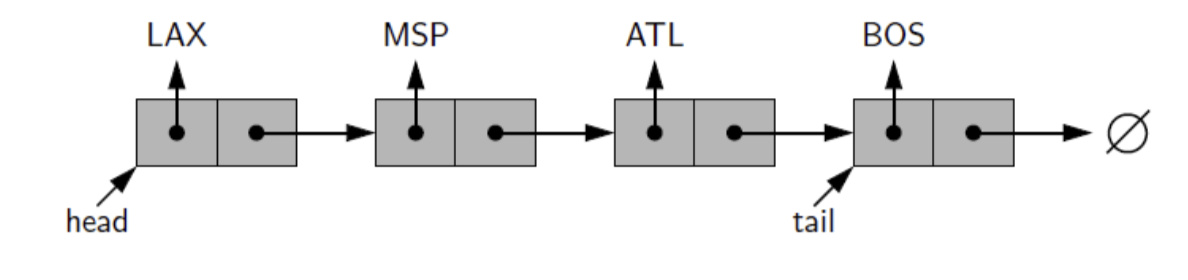4) Inserting an Element at the Head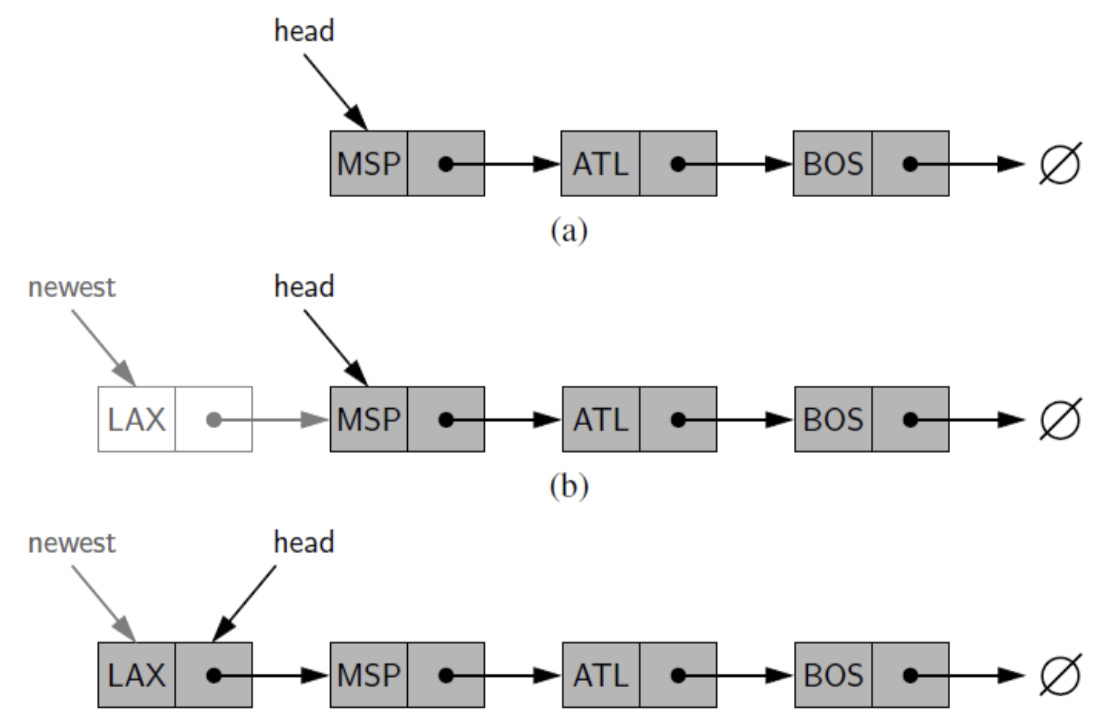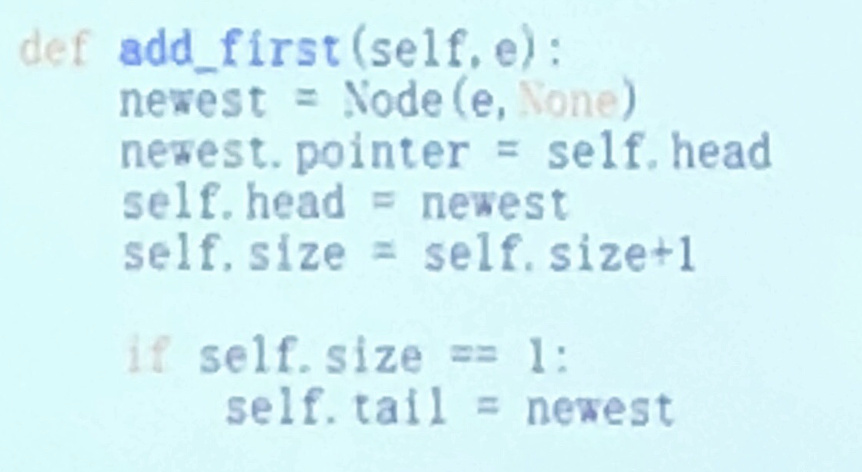5) Inserting an Element at the Tail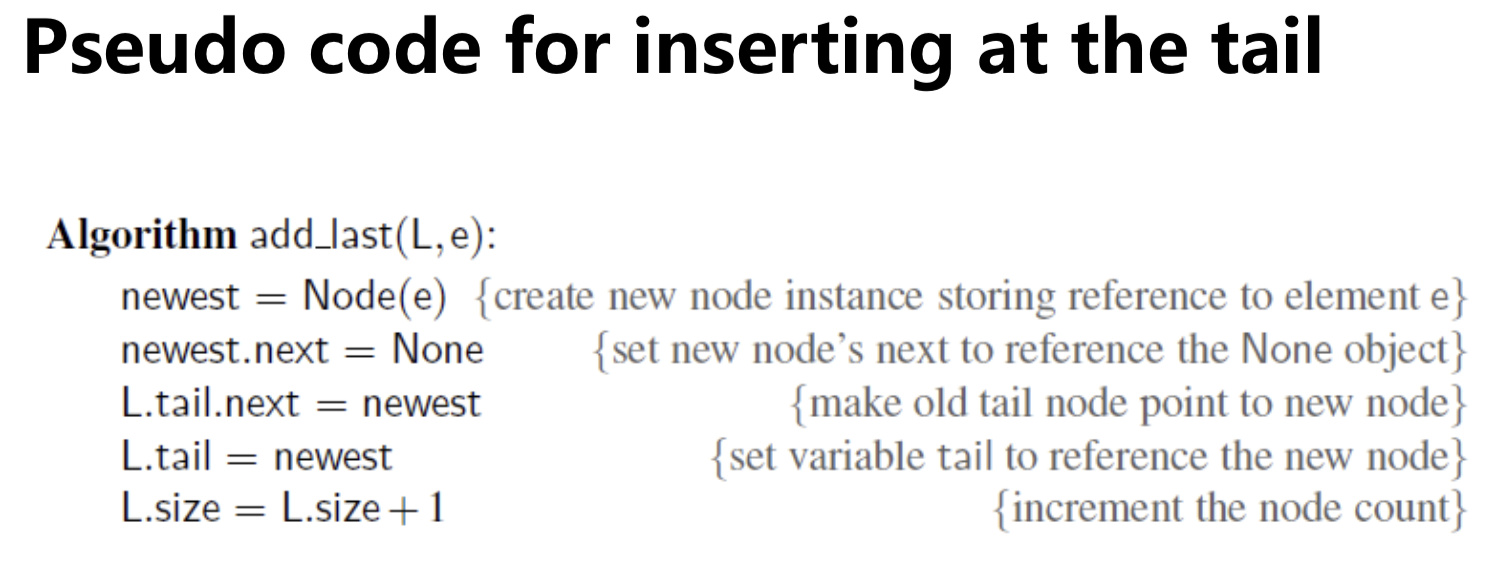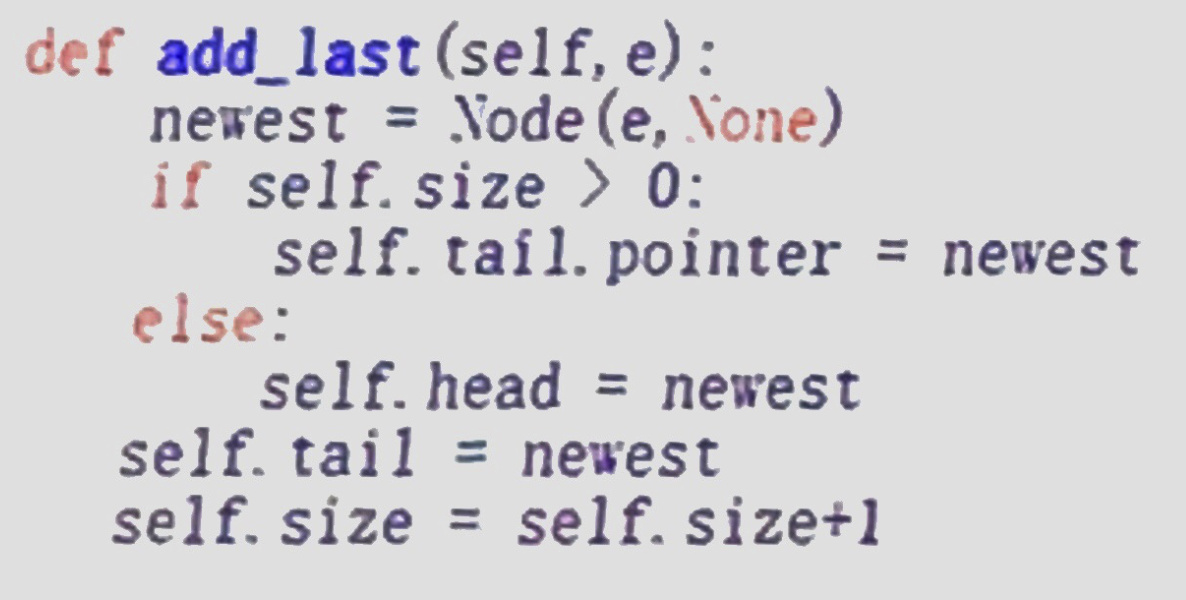6) Removing an Element from the head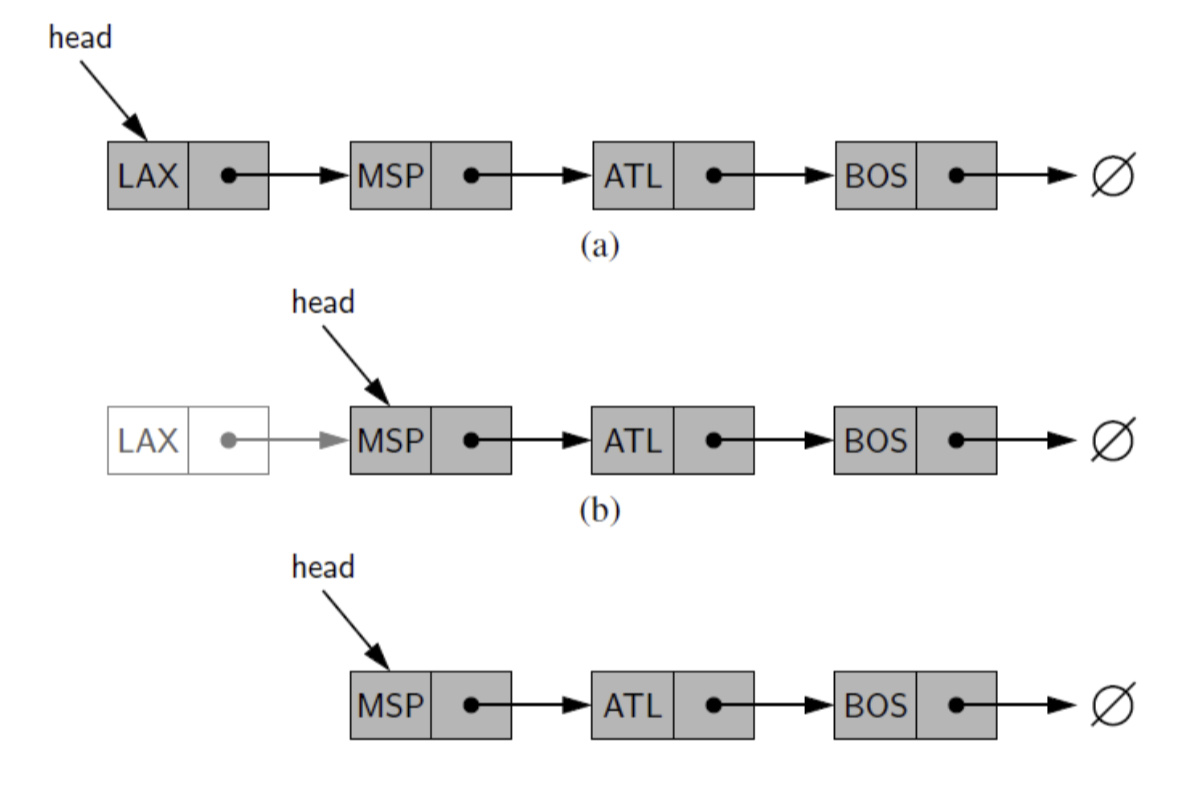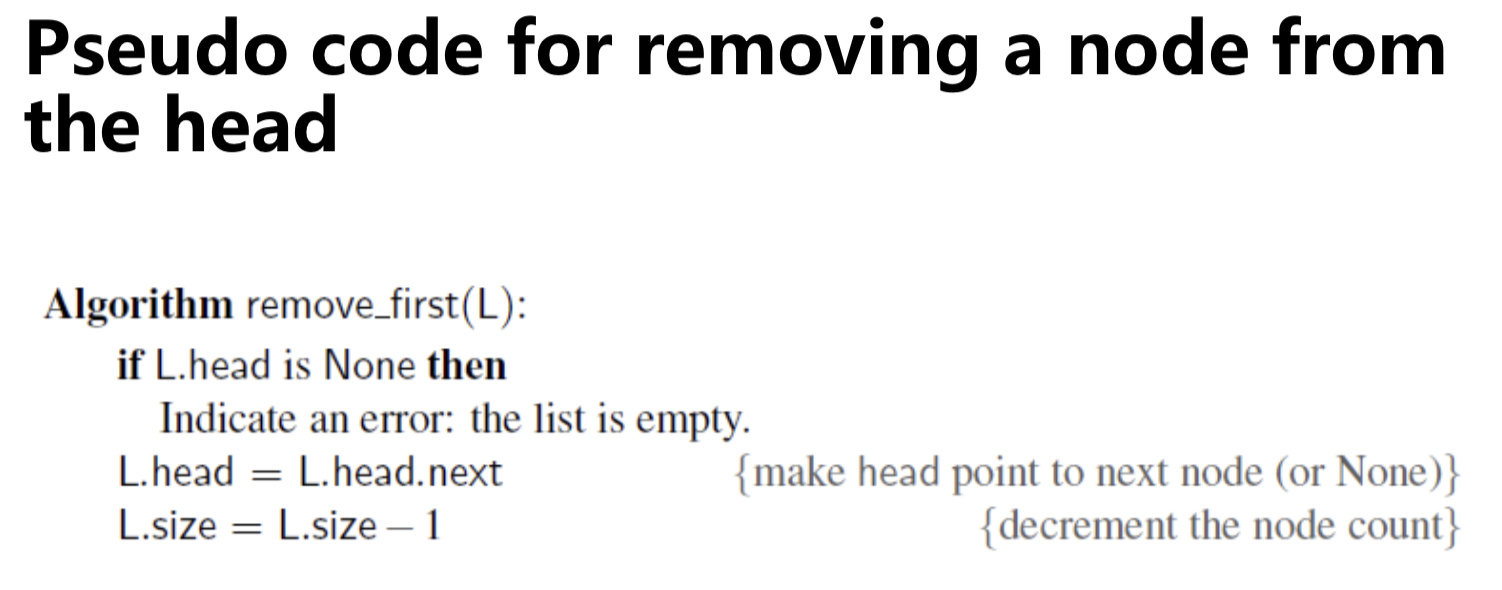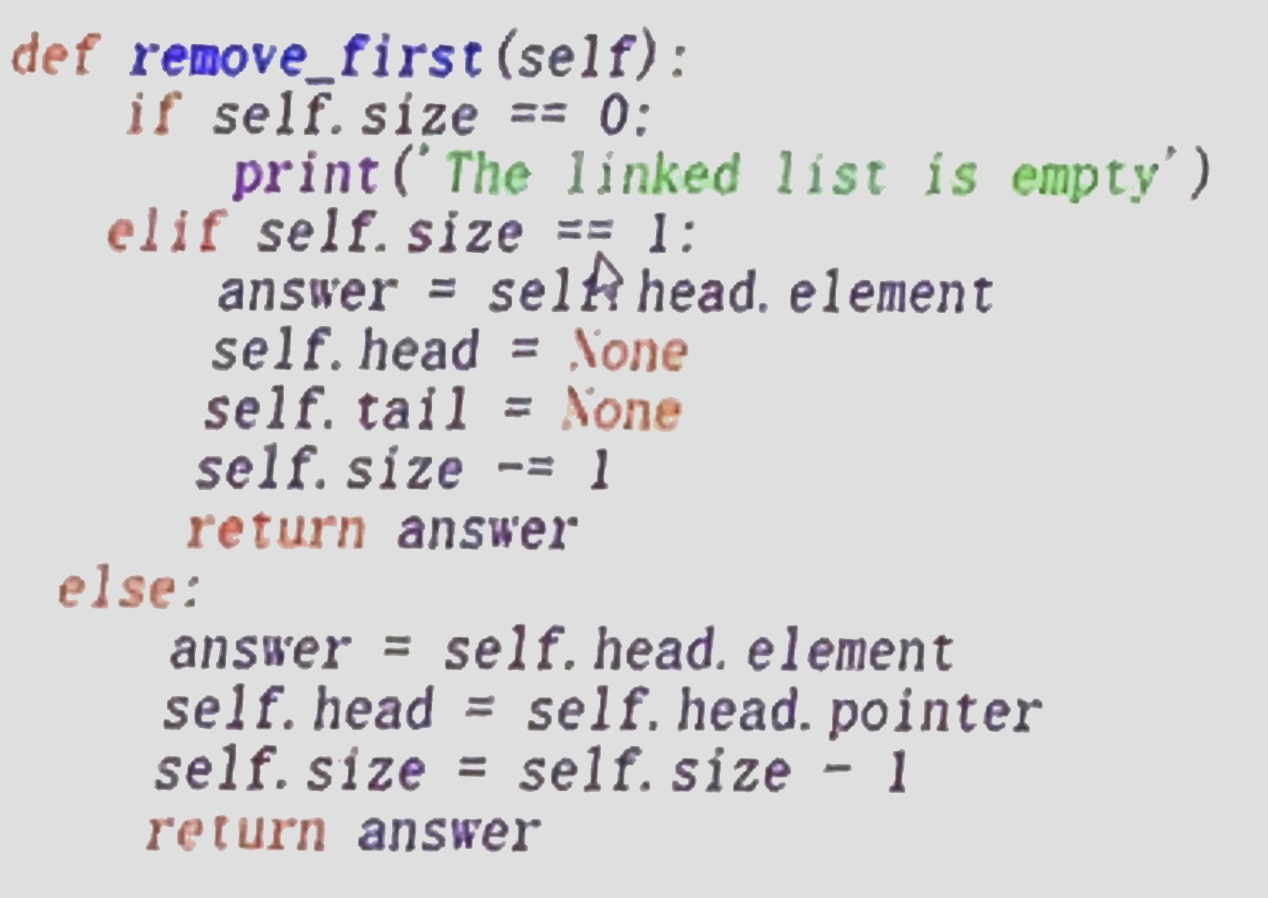7) code

8) Practice

1. Implement stack with a singly linked list

1. Implement queue with a singly linked list

### 2.4 Circularly Linked List 循环链表

• The tail of a linked list can use its next reference to point back to the head of the list
• Such a structure is usually called a circularly linked list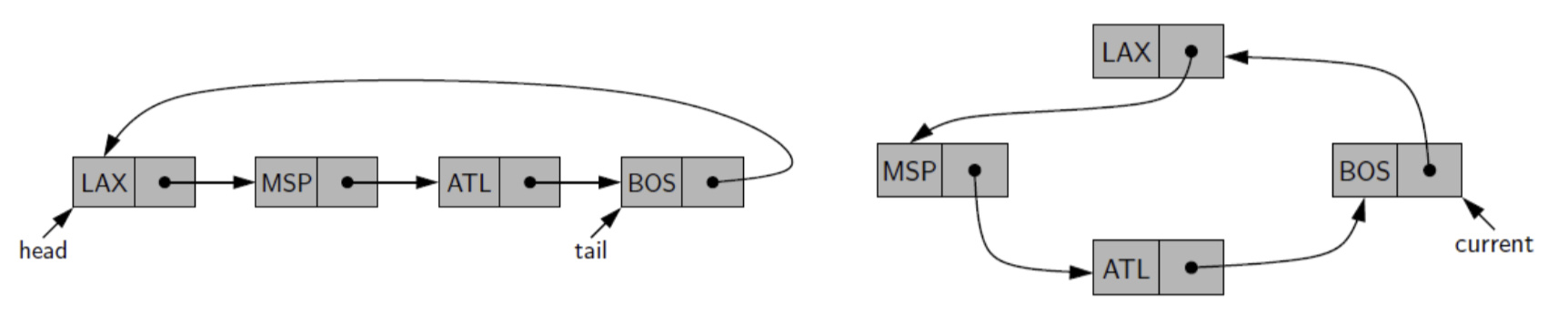1) Example: Round-robin scheduler 循环调度

multi-tasking

• A round-robin scheduler iterates through a collection of elements in a circular fashion and “serves” each element by performing a given action on it
• Such a scheduler is used, for example, to fairly allocate a resource that must be shared by a collection of clients
• For instance, round-robin scheduling is often used to allocate slices of CPU time to various applications running concurrently on a computer

2) Implementing round-robin scheduler using standard queue

• A round-robin scheduler could be implemented with the standard queue, by repeatedly performing the following steps on queue Q:

1. e = Q.dequeue()
2. Service element e
3. Q.enqueue(e)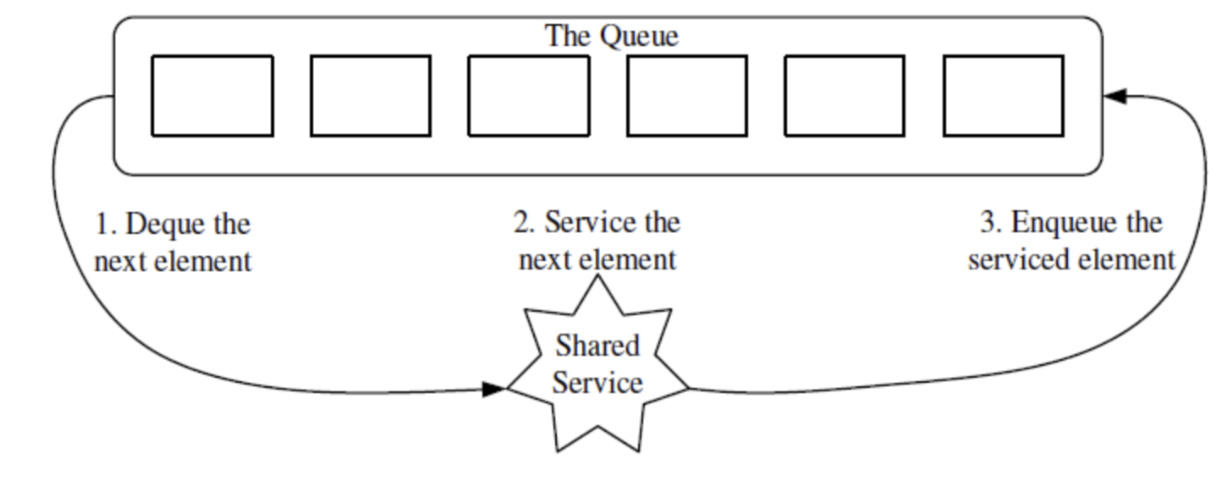3) Implement a Queue with a Circularly Linked List### 2.5 Doubly linked list 双链表

• For a singly linked list, we can efficiently insert a node at either end of a singly linked list, and can delete a node at the head of a list
• But we cannot efficiently delete a node at the tail of the list

• We can define a linked list in which each node keeps an explicit
reference to the node before it and a reference to the node after it

• In order to avoid some special cases when operating near the boundaries of a doubly linked list, it helps to add special nodes at
both ends of the list: a header node at the beginning of the list, and a trailer node at the end of the list
• These “dummy” nodes are known as sentinels (or guards), and they do not store elements of the primary sequence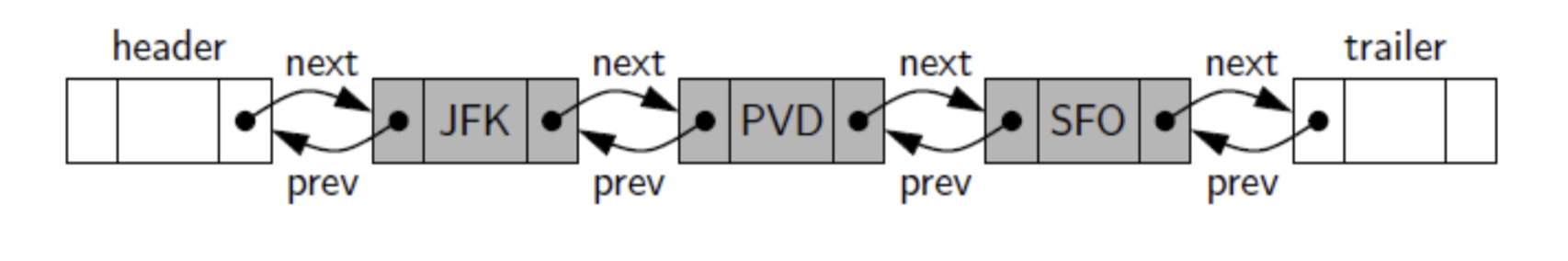Code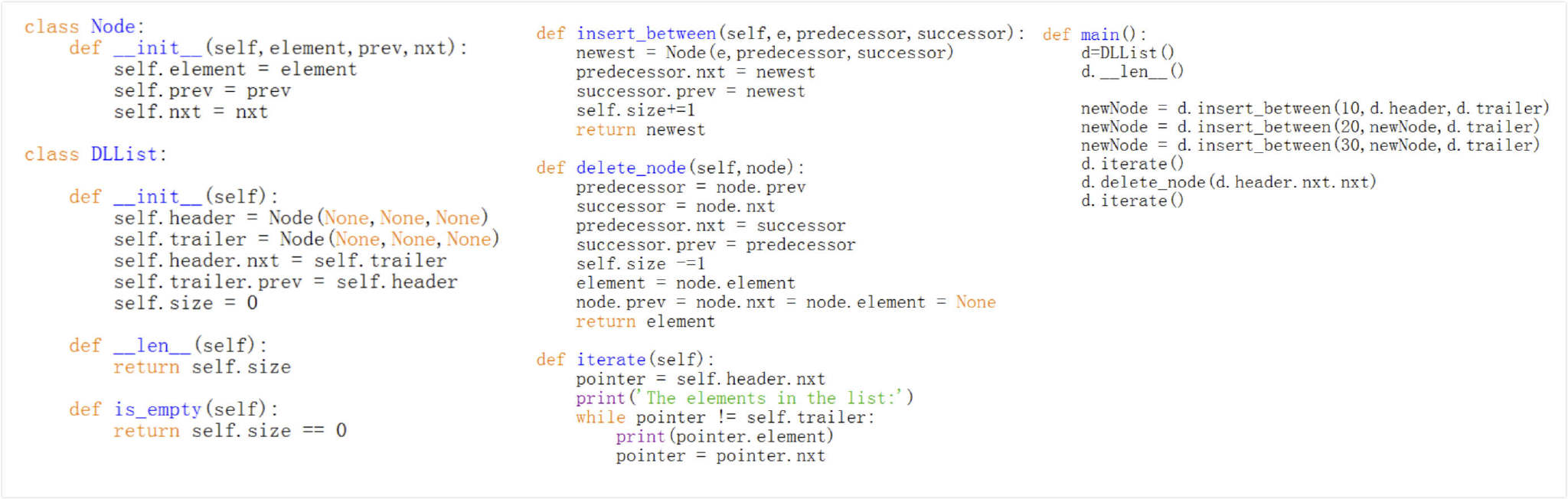1) Inserting in the middle of a doubly linked list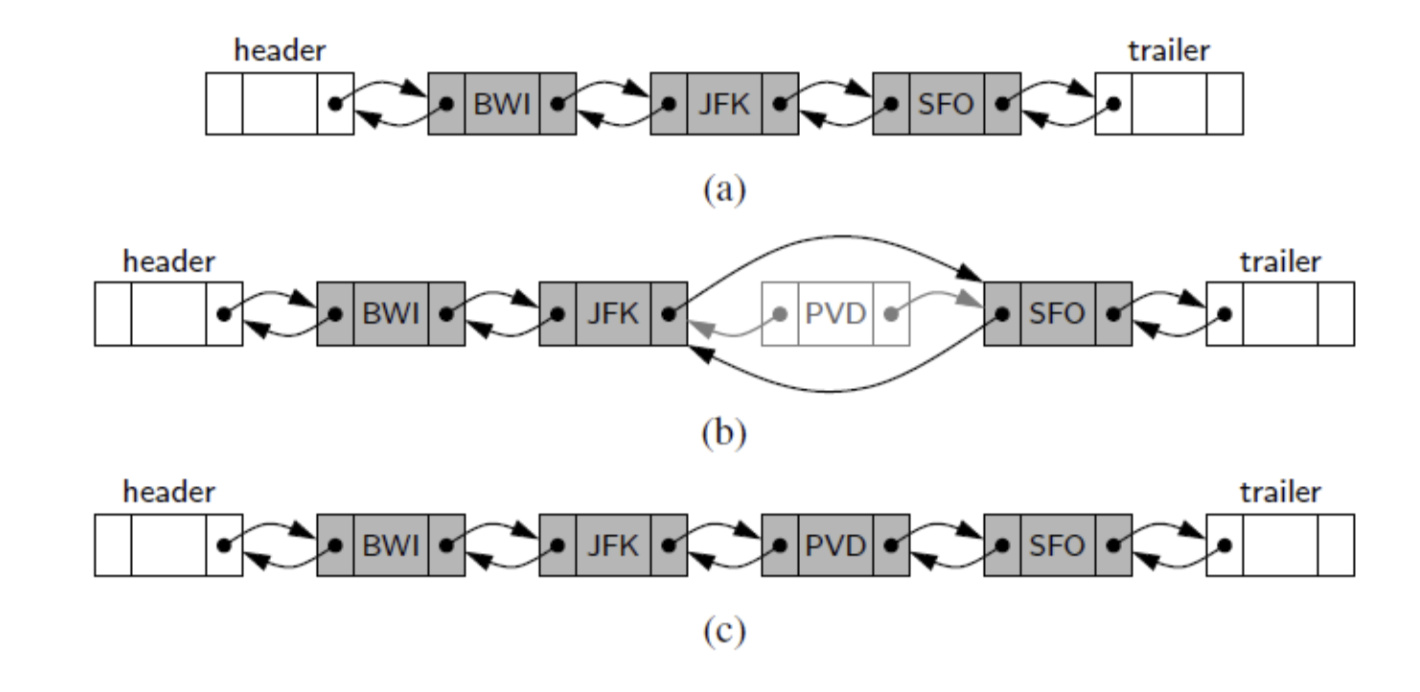2) Deleting from the doubly linked list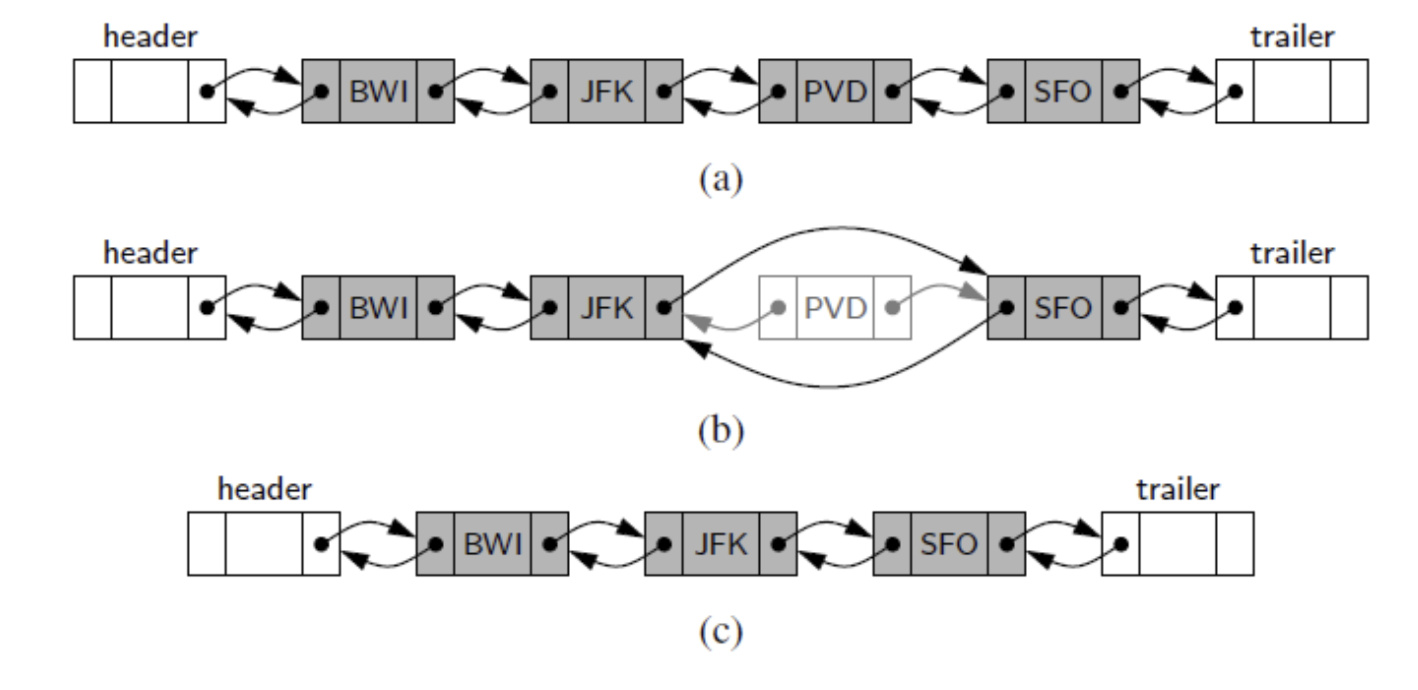### 2.6 Tree 树

• A tree is a data structure that stores elements hierarchically（分层的）
• With the exception of the top element, each element in a tree has a parent element and zero or more children elements
• We typically call the top element the root of the tree（根节点）, but it is drawn as the highest element1) Edge 边 and path 路径

• An edge 边 of tree T is a pair of nodes (u,v) such that u is the parent of v, or vice versa
• A path 路径 of T is a sequence of nodes such that any two consecutive nodes in the sequence form an edge
• The depth 深度 of a node v is the length of the path connecting root node and v（不包括自己）
• leaf node 叶节点
• internal node 内部节点

2) Ordered tree

A tree is ordered if there is a meaningful linear order among the children of each node（树中每个结点的各子树看成是从左到右有次序的）; such an order is usually visualized by arranging siblings from left to right, according to their order （之前公司的结构就是 Unordered Tree）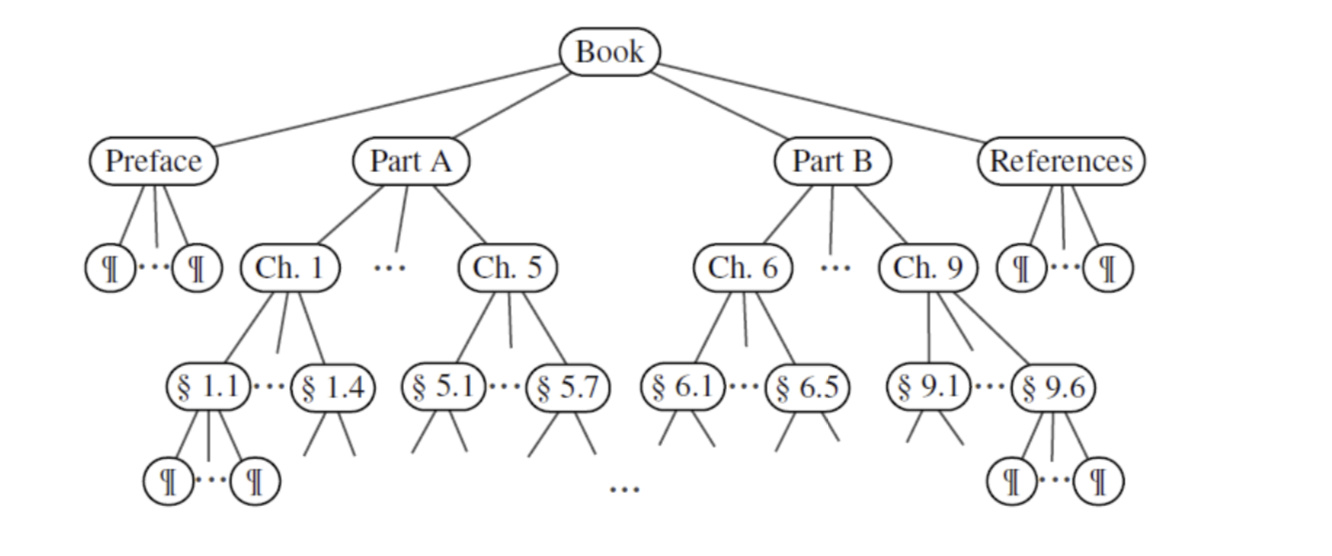3) Binary tree

A binary tree is an ordered tree with the following properties:

1. Every node has at most two children
2. Each child node is labeled as being either a left child or a right child
3. A left child precedes a right child in the order of children of a node

The subtree rooted at a left or right child of an internal node v is called a left subtree or right subtree, respectively, of v

A binary tree is proper if each node has either zero or two children（要不没有，要不2个）. Some people also refer to such trees as being full binary trees

4) Example: Represent an expression with binary tree

An arithmetic expression（算术表达式）can be represented by a binary tree whose leaves are associated with variables or constants, and whose internal nodes are associated with one of the operators +, −, ×, and /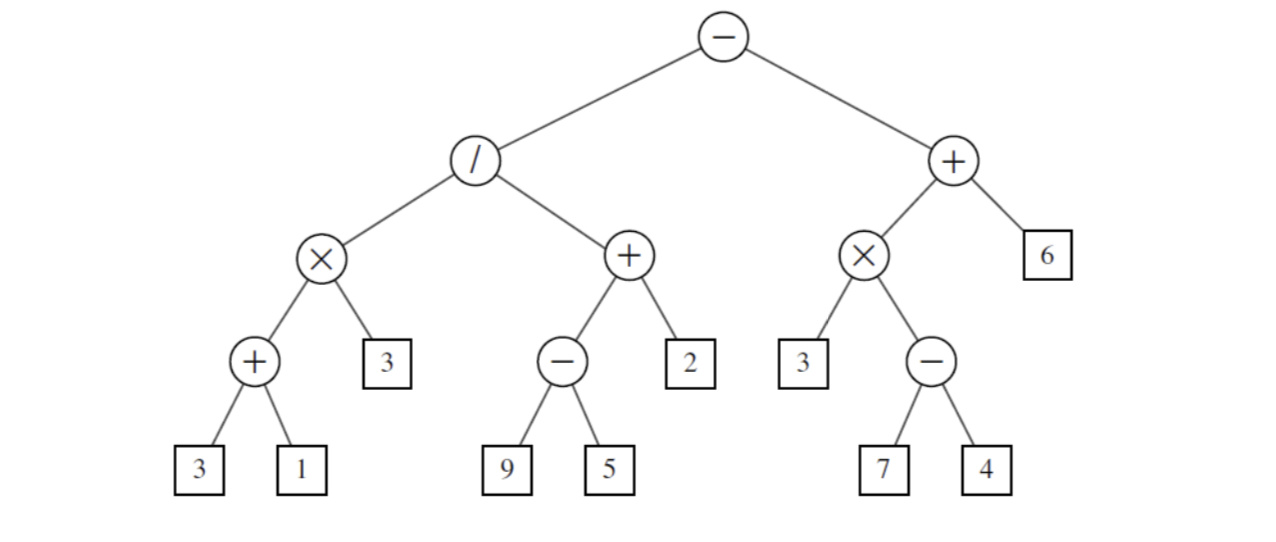5) Binary tree class

• We define a tree class based on a class called Node; an element is stored as a node

• Each node contains 3 references（像是四个格子，第一个是数据）

1. one pointing to the parent node,
2. two pointing to the child nodes

6) Implementing the binary tree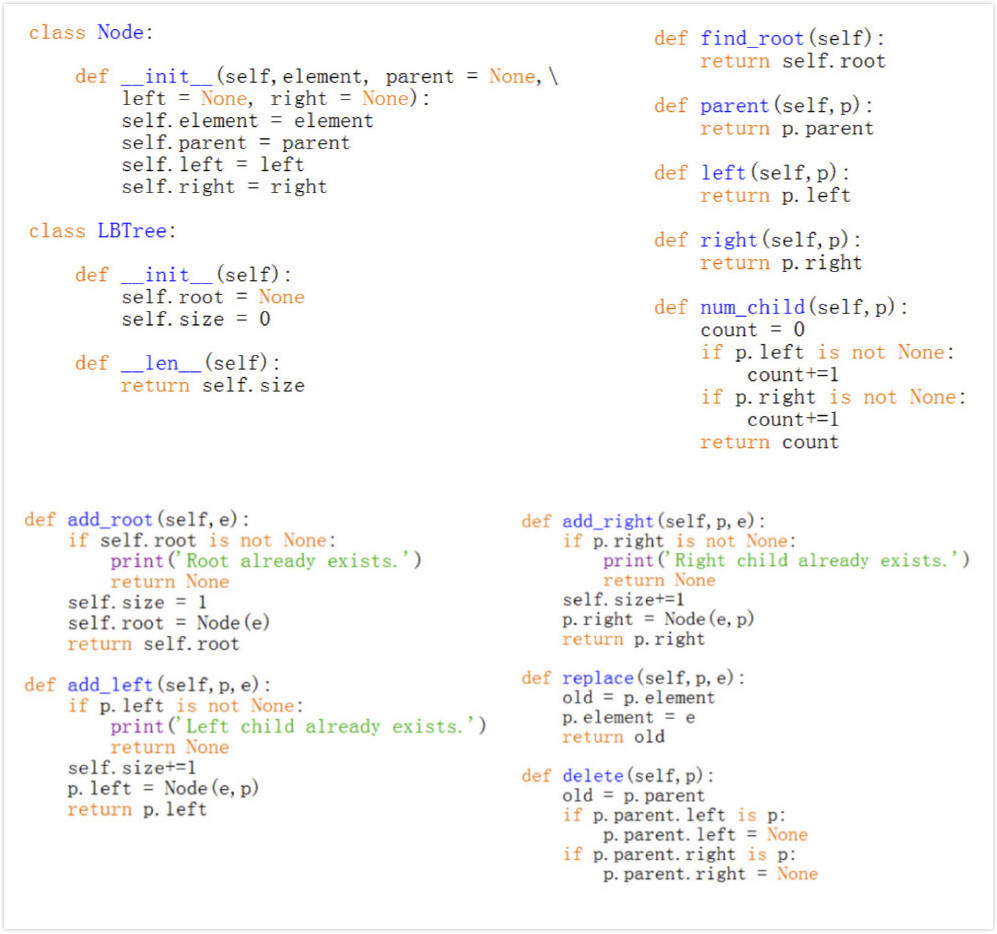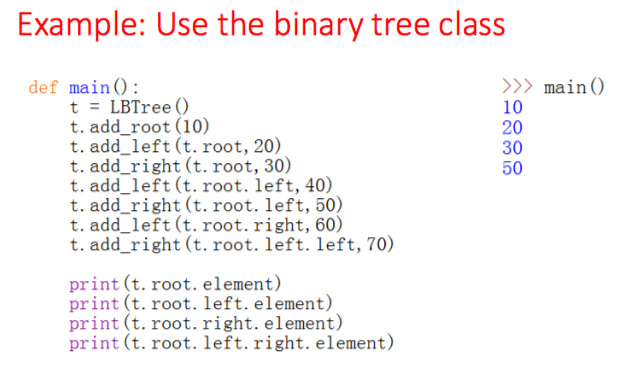7) Depth first search (DFS) 深度优先搜索算法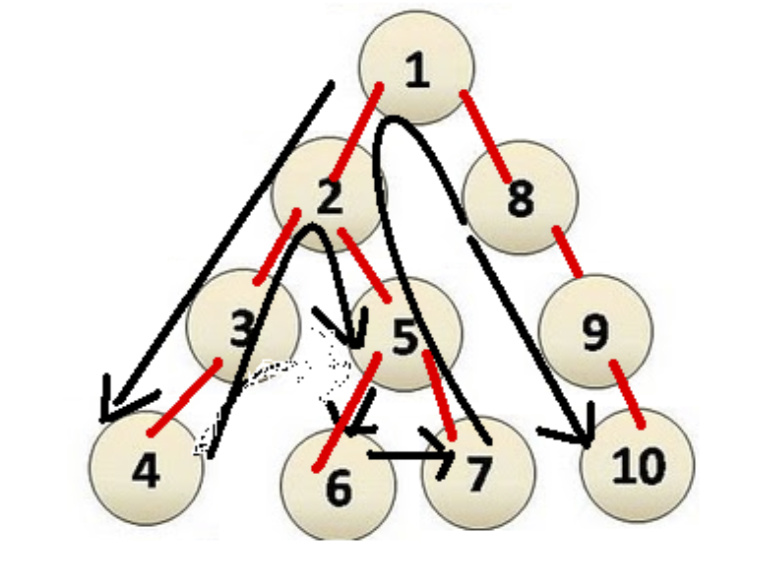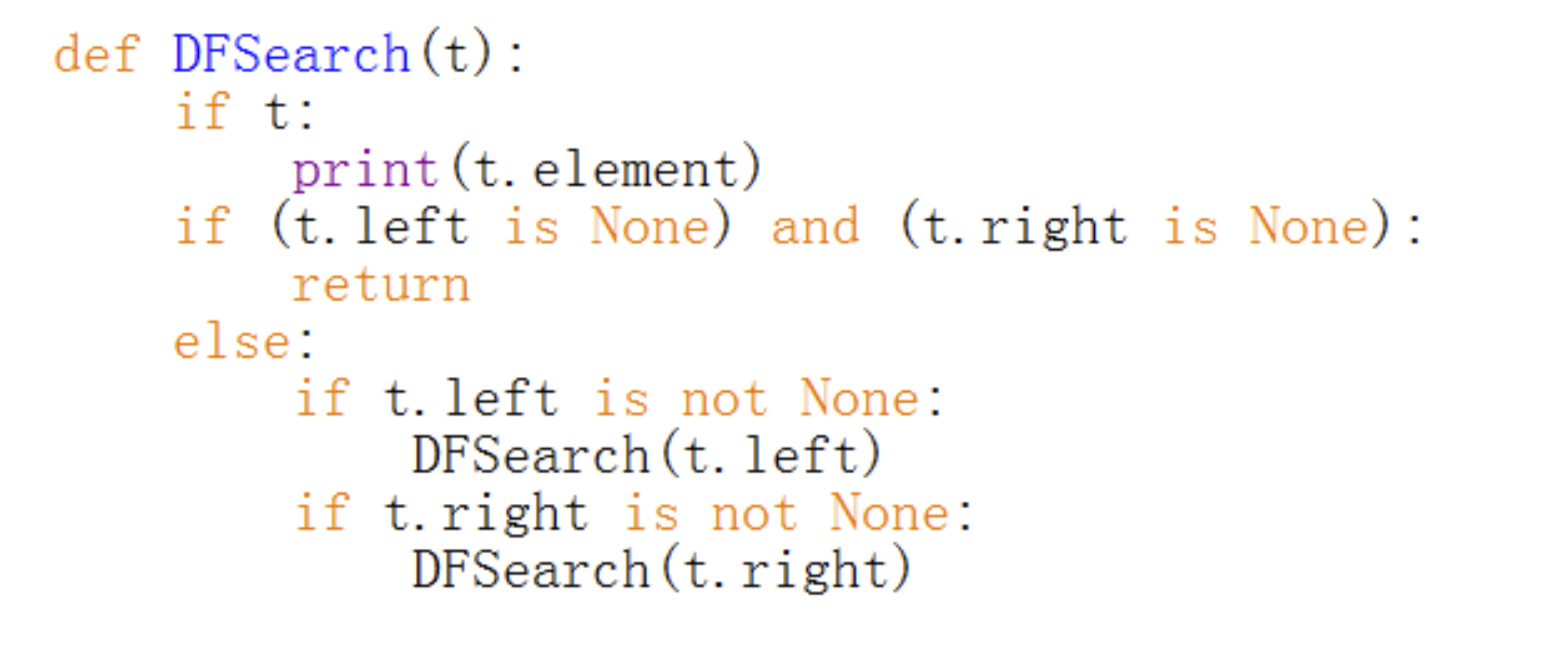8) Breadth first search 广度优先搜索

Starts at the root and we visit all the positions at depth d before we visit the positions at depth d +1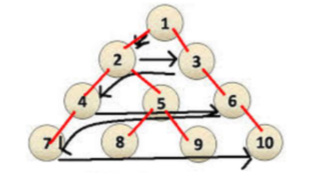Example: finding the best strategy in a game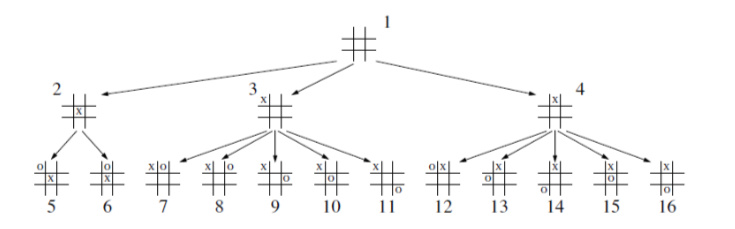Code